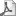# HetMet Extensions for GHC

This page describes and provides a branch of GHC which facilitates heterogeneous metaprogramming by adding three features:

• GHC.HetMet.GArrow, a set of type classes for generalized arrows; guest languages are created by implementing instances of these classes.

• Support for modal types inhabited by multi-level terms via the new -XModalTypes flag; guest programs are created by writing expressions of a boxed type.

• A new compiler pass which translates guest programs from multi-level terms into GArrow-instance-polymorphic one-level terms. This exploits the one-to-one correspondence between multi-level languages and generalized arrows. Roughly speaking, terms of modal type g(a->b) become ordinary Haskell terms of type GArrow g => (g a b). The new pass runs after the desugarer.

Collectively, these three features – none of which is very useful without the other two – are known as the heterogeneous metaprogramming (“HetMet”) extensions for GHC.

## What are Generalized Arrows?

Like Haskell Arrows, generalized arrows provide a platform for metaprogramming. Unlike multi-stage languages and Haskell Arrows, generalized arrows allow for heterogeneous metaprogramming. Haskell Arrows support metaprogramming only when the guest language is a superset of Haskell, because every Haskell function can be promoted to a guest language expression using arr.

Generalized arrows remove the assumption that this sort of promotion is possible. This enables heterogeneous metaprogramming.

Here's what the type class looks like:

class Category g => GArrow g (**) u where
--id         :: g x x
--comp       :: g x y -> g y z -> g x z
ga_first     :: g x y -> g (x ** z) (y ** z)
ga_second    :: g x y -> g (z ** x) (z ** y)
ga_cancell   :: g (u**x) x
ga_cancelr   :: g (x**u) x
ga_uncancell :: g x       (u**x)
ga_uncancelr :: g x       (x**u)
ga_assoc     :: g ((x**y)**z) (x**(y**z))
ga_unassoc   :: g (x**(y**z)) ((x**y)**z)

The following methods are provided by useful subclasses of GArrow; they account for languages with weakening, exchange, contraction, and recursion:

class GArrow g (**) u => GArrowDrop g (**) u where
ga_drop      :: g x u

class GArrow g (**) u => GArrowCopy g (**) u where
ga_copy      :: g x (x**x)

class GArrow g (**) u => GArrowSwap g (**) u where
ga_swap      :: g (x**y) (y**x)

class GArrow g (**) u => GArrowLoop g (**) u where
ga_loop      :: g (x**z) (y**z) -> g x y

The above five type classes are the only ones which are known to the compiler and given special status.

Every Haskell Arrow is a GArrow. Just like Haskell Arrows (and monads), generalized arrows can be used to generate programs in guest languages with features that Haskell lacks. For example, mutable state can be used to produce sequential circuits even though Haskell programs cannot have side effects.

## Implementation

The new compiler pass is implemented in Coq, using a complete formalization ofSystem FC1. Details are here. The flattening transformation relies on the fact that Haskell terms form a category and also that Haskell typing proofs form a category; these facts use my library for category theory in Coq.

## More Information

• There is a tutorial program you can play with, though you'll need to build the compiler using the instructions above. The tutorial is here.